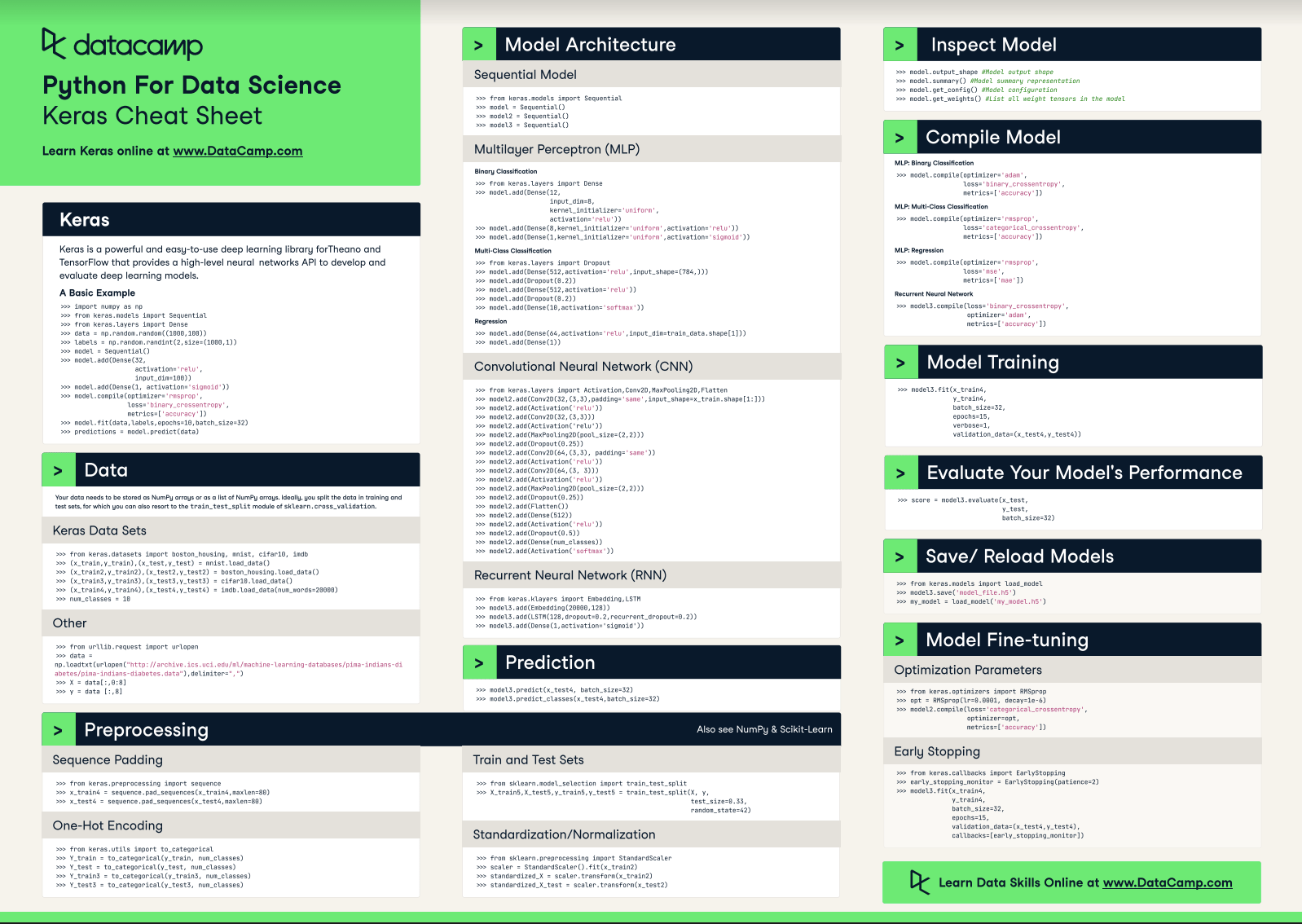Official Blog
python
+4

# Keras Cheat Sheet: Neural Networks in Python

Make your own neural networks with this Keras cheat sheet to deep learning in Python for beginners, with code samples.

Keras is an easy-to-use and powerful library for Theano and TensorFlow that provides a high-level neural networks API to develop and evaluate deep learning models.

We recently launched one of the first online interactive deep learning course using Keras 2.0, called "Deep Learning in Python".

Now, DataCamp has created a Keras cheat sheet for those who have already taken the course and that still want a handy one-page reference or for those who need an extra push to get started.

In no time, this Keras cheat sheet will make you familiar with how you can load datasets from the library itself, preprocess the data, build up a model architecture, and compile, train, and evaluate it. As there is a considerable amount of freedom in how you build up your models, you'll see that the cheat sheet uses some of the simple key code examples of the Keras library that you need to know to get started with building your own neural networks in Python.

Furthermore, you'll also see some examples of how to inspect your model, and how you can save and reload it. Lastly, you’ll also find examples of how you can predict values for test data and how you can fine tune your models by adjusting the optimization parameters and early stopping.

In short, you'll see that this cheat sheet not only presents you with the six steps that you can go through to make neural networks in Python with the Keras library.In short, this cheat sheat will boost your journey with deep learning in Python: you'll have preprocessed, created, validated and tuned your deep learning models in no time thanks to the code examples!

## Python For Data Science Cheat Sheet: Keras

Keras is a powerful and easy-to-use deep learning library for Theano and TensorFlow that provides a high-level neural networks API to develop and evaluate deep learning models.

### A Basic Example

>>> import numpy as np
>>> from keras.models import Sequential
>>> from keras.layers import Dense
>>> data = np.random.random((1000,100))
>>> labels = np.random.randint(2,size=(1000,1))
>>> model = Sequential()
>>> model.compile(optimizer='rmsprop', loss='binary_crossentropy', metrics=['accuracy'])
>>>  model.fit(data,labels,epochs=10,batch_size=32)
>>>  predictions = model.predict(data)

### Data

Your data needs to be stored as NumPy arrays or as a list of NumPy arrays. Ideally, you split the data in training and test sets, for which you can also resort to the train_test_split module of sklearn.cross_validation.

#### Keras Data Sets

>>> from keras.datasets import boston_housing, mnist, cifar10, imdb
>>> num_classes = 10

#### Other

>>> from urllib.request import urlopen
>>> X = data[:,0:8]
>>> y = data [:,8]

### Preprocessing

>>> from keras.preprocessing import sequence
>>> x_test4 = sequence.pad_sequences(x_test4,maxlen=80)

#### One-Hot Encoding

>>> from keras.utils import to_categorical
>>> Y_train = to_categorical(y_train, num_classes)
>>> Y_test = to_categorical(y_test, num_classes)
>>> Y_train3 = to_categorical(y_train3, num_classes)
>>> Y_test3 = to_categorical(y_test3, num_classes)

#### Train And Test Sets

>>> from sklearn.model_selection import train_test_split
>>> X_train5, X_test5, y_train5, y_test5 = train_test_split(X, y, test_size=0.33, random_state=42)

### Standardization/Normalization

>>> from sklearn.preprocessing import StandardScaler
>>> scaler = StandardScaler().fit(x_train2)
>>> standardized_X = scaler.transform(x_train2)
>>> standardized_X_test = scaler.transform(x_test2)

### Model Architecture

#### Sequential Model

>>> from keras.models import Sequential
>>> model = Sequential()
>>> model2 = Sequential()
>>> model3 = Sequential()

#### Multi-Layer Perceptron (MLP)

Binary Classification

>>> from keras.layers import Dense
>>> model.add(Dense(1, kernel_initializer='uniform', activation='sigmoid'))

Multi-Class Classification

>>> from keras.layers import Dropout
>>> model.add(Dense(10,activation='softmax'))

Regression

>>> model.add(Dense(64, activation='relu', input_dim=train_data.shape))
>>> model.add(Dense(1))

#### Convolutional Neural Network (CNN)

>>> from keras.layers import Activation, Conv2D, MaxPooling2D, Flatten
>>> model2.add(Activation('softmax'))

#### Recurrent Neural Network (RNN)

>>> from keras.klayers import Embedding,LSTM
>>> model3.add(Dense(1,activation='sigmoid'))

### Inspect Model

Model output shape

>>> model.output_shape

Model summary representation

>>> model.summary()

Model configuration

>>> model.get_config()

List all weight tensors in the model

>>> model.get_weights()

### Compile Model

#### Multi-Layer Perceptron (MLP)

MLP: Binary Classification

>>> model.compile(optimizer='adam', loss='binary_crossentropy', metrics=['accuracy'])

MLP: Multi-Class Classification

>>> model.compile(optimizer='rmsprop', loss='categorical_crossentropy', metrics=['accuracy'])

MLP: Regression

>>> model.compile(optimizer='rmsprop', loss='mse', metrics=['mae'])

#### Recurrent Neural Network (RNN)

>>> model3.compile(loss='binary_crossentropy', optimizer='adam', metrics=['accuracy'])

### Model Training

>>> model3.fit(x_train4, y_train4, batch_size=32, epochs=15, verbose=1, validation_data=(x_test4, y_test4))

>>> score = model3.evaluate(x_test, y_test, batch_size=32)

### Prediction

>>> model3.predict(x_test4, batch_size=32)
>>> model3.predict_classes(x_test4,batch_size=32)

>>> from keras.models import load_model
>>> model3.save('model_file.h5')
>>> my_model = load_model('my_model.h5')

### Model Fine-Tuning

#### Optimization Parameters

>>> from keras.optimizers import RMSprop
>>> opt = RMSprop(lr=0.0001, decay=1e-6)
>>> model2.compile(loss='categorical_crossentropy', optimizer=opt, metrics=['accuracy'])

#### Early Stopping

>>> from keras.callbacks import EarlyStopping
>>> early_stopping_monitor = EarlyStopping(patience=2)
>>> model3.fit(x_train4, y_train4, batch_size=32, epochs=15, validation_data=(x_test4, y_test4), callbacks=[early_stopping_monitor])

### Going Further

Begin with our Keras tutorial for beginners, in which you'll learn in an easy, step-by-step way how to explore and preprocess the wine quality data set, build up a multi-layer perceptron for classification and regression tasks, compile, fit and evaluate the model and fine-tune the model that you have built.

Also, don't miss out on our Scikit-Learn cheat sheet, NumPy cheat sheet and Pandas cheat sheet!